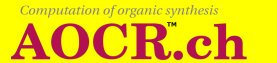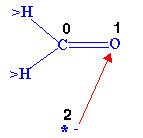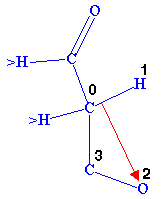# 1. Organic chemistry basics

### 1.3. Reaction step rules

A reaction proceeds in steps and each step has its own mechanism. The mechanism of a step is represented by a reaction step rule containing a pattern to be matched by a corresponding image in a formula and the instructions for the movement of the corresponding bonds, charges or electrons.

In a pattern, which is a more general representation than the representation given by a formula, expressions are possibly connected by lines and are possibly carrying a number of positive or negative charges and of electrons. The instructions are given by arrows between lines, expressions, charges and electrons.

Subsection 3.1.2 explains how to build a reaction step rule with the Graphic Composer.

The reaction step rule instructions are applied to a formula whenever there is a matching correspondence between the expressions of the rule and the symbols in the formula. Every application of a rule preserves the symbols as well as their valence (the sum of the values of its bonds to another symbol increased by the number of charges and electrons).

For example the reaction in Figure 1.2 can be seen, to proceed in two steps. First the ion adds to the carbonyl of the other molecule, then the resulting compound eliminates OH-. The corresponding reaction step rules are represented in the Figures 1.4, 1.5.
 Figure 1.4Figure 1.5In a matching correspondence a symbol greater than H is assigned to >H, any symbol can correspond to *. No bond, a single bond, a double bond must correspond to no bond, a single bond, a double bond respectively. When the rule in Figure 1.4 is applied, the double bond between the symbols corresponding to 0,1 becomes a single bond, the charge on the symbol corresponding to 2 moves to the correspondent of 1 and finally a single bond is formed between the symbols corresponding to 3,0. When the rule in Figure 1.5 is applied, the bond between the correspondents of 0,1 is broken, a new bond forms between the corresponding symbols of 1,2, again the bond between the correspondents of 2,3 is broken and finally the single bond between the correspondents of 0,3 becomes a double bond.